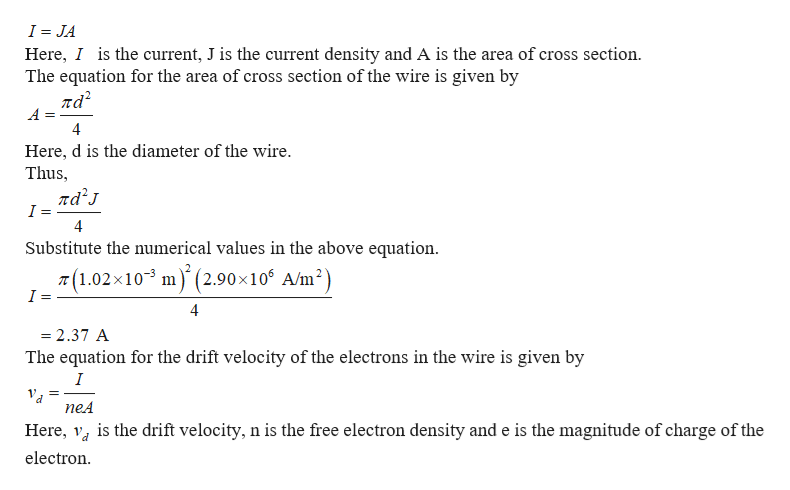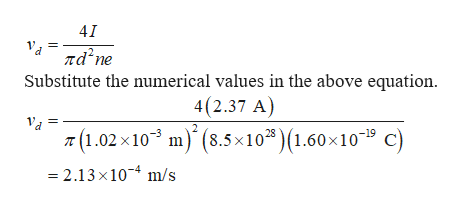# An 18 gauge copper wire (diameter 1.02 mm) carries a current with a current density of 2.90×10^6 A/m2. The density of free electrons for copper is 8.5×10^28 electrons per cubic meter.Calculate the current in the wire.Express your answer with the appropriate units.Calculate the magnitude of the drift velocity of electrons in the wire.Express your answer with the appropriate units.

Question
61 views

An 18 gauge copper wire (diameter 1.02 mm) carries a current with a current density of 2.90×10^6 A/m2. The density of free electrons for copper is 8.5×10^28 electrons per cubic meter.

Calculate the current in the wire.
Calculate the magnitude of the drift velocity of electrons in the wire.
check_circle

Step 1

The equation for the current in the wire is given byhelp_outlineImage TranscriptioncloseI JA Here, I is the current, J is the current density and A is the area of cross section The equation for the area of cross section of the wire is given by ла? 4 Here, d is the diameter of the wire. Thus ла'л I 4 Substitute the numerical values in the above equation T(1.02x103 m(2.90 x 10 A/m2) I = 4 = 2.37 A The equation for the drift velocity of the electrons in the wire is given by I neA is the drift velocity, n is the free electron density and e is the magnitude of charge of the Here, electron fullscreen
Step 2

Putting the equatio...help_outlineImage Transcriptionclose41 nd'ne Substitute the numerical values in the above equation. 4(2.37 A) (1.02x10 m) (8.5x102) (1.60 x10" C) 2.13x10 m/s fullscreen

### Want to see the full answer?

See Solution

#### Want to see this answer and more?

Solutions are written by subject experts who are available 24/7. Questions are typically answered within 1 hour.*

See Solution
*Response times may vary by subject and question.
Tagged in

### Semiconductors